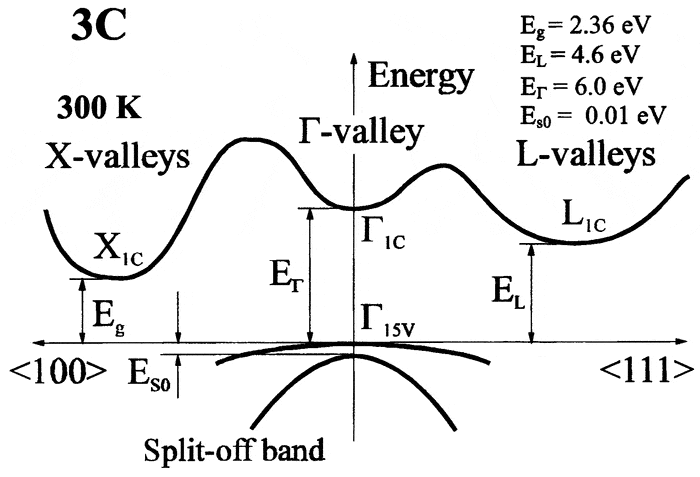# Significance of band gap in k-space

I am struggling in understanding what the band structure (E vs. k) diagram tells me.

Let's say I have the following material:
http://www2.warwick.ac.uk/fac/sci/p...s/indirect_absorption/indirect_absorption.bmp

Let the direct band gap be denoted as ωg. Let's say the temperature is such that it is possible for energies up to ħ(ω+Ω). But since ε(ħ(ω+Ω))<ε(ωg), can I say that I will only observe appreciable current when I apply a electric field in the direction of +kr?

ZapperZ
Staff Emeritus
There is something weird about that diagram. ħω is an energy transition, but the lateral transition is a MOMENTUM transition, not energy. So the units/dimensions are all wrong.

In an indirect transition, you need a momentum change to achieve that transition. So just simply adding energy, such as in the form of heat, will not achieve such a transition. It will require a momentum transfer, usually from phonon exchange, to provide such a momentum transition.

All your band diagram is showing is that the conduction band minimum is not at the same momentum vector as the valence band maximum. So to cause a transition from the valence band maximum to the conduction band minimum, you need energy and momentum change.

Zz.

Cthugha
There is something weird about that diagram. ħω is an energy transition, but the lateral transition is a MOMENTUM transition, not energy. So the units/dimensions are all wrong.

It is a standard diagram for indirect absorption assisted by phonon emission, but it should never ever be quoted out of context like here. The photon energy $\hbar \omega$ equals the sum of the indirect band gap energy and the phonon energy $\hbar \Omega$. The photon essentially contributes all the energy and negligible momentum. The phonon essentially contributes all the momentum and negligible energy. The momenta are not shown explicitly, but are explained in the accompanying text That would have been clear if the OP had cited the whole page including the explanation instead of just the picture.

Let the direct band gap be denoted as ωg. Let's say the temperature is such that it is possible for energies up to ħ(ω+Ω). But since ε(ħ(ω+Ω))<ε(ωg), can I say that I will only observe appreciable current when I apply a electric field in the direction of +kr?

That is a bit puzzling. You seem to be interested in questions of transport (current and conductivity and stuff), while the diagram is mainly of interest when discussing the indirect band gap from an optics point of view. As ZapperZ already pointed out, phonon assisted processes are already sufficient for realizing such an indirect transmission.

Please note that the main interesting thing about indirect semiconductors is something different. If you have enough thermal energy for electrons to make the direct band gap transition, but there is an indirect band gap of lower energy nearby in momentum space, electrons in the conduction band will relax to that energy minimum of the conduction band quite quickly. From there, they cannot directly recombine and go back to the valence band just by photon emission. An additional phonon is required. As these phonon assisted processes are way more improbable than the direct transition, the carrier lifetimes are typically much larger in indirect band gap semiconductors like silicon compared to semiconductors with a direct band gap.

Thank you for the responses. It helped clarified some doubts which I had about the concept.

I asked this question to my professor (albeit in less detail) last semester when I had my intro to solid state physics course but he kinda gave the 'I don't know where you are coming from' look so I did not probe further. I think I understand where this look he is having comes from now.

I hope I am not complicating the problem too much by shifting our attention to yet another band structure (SiC):